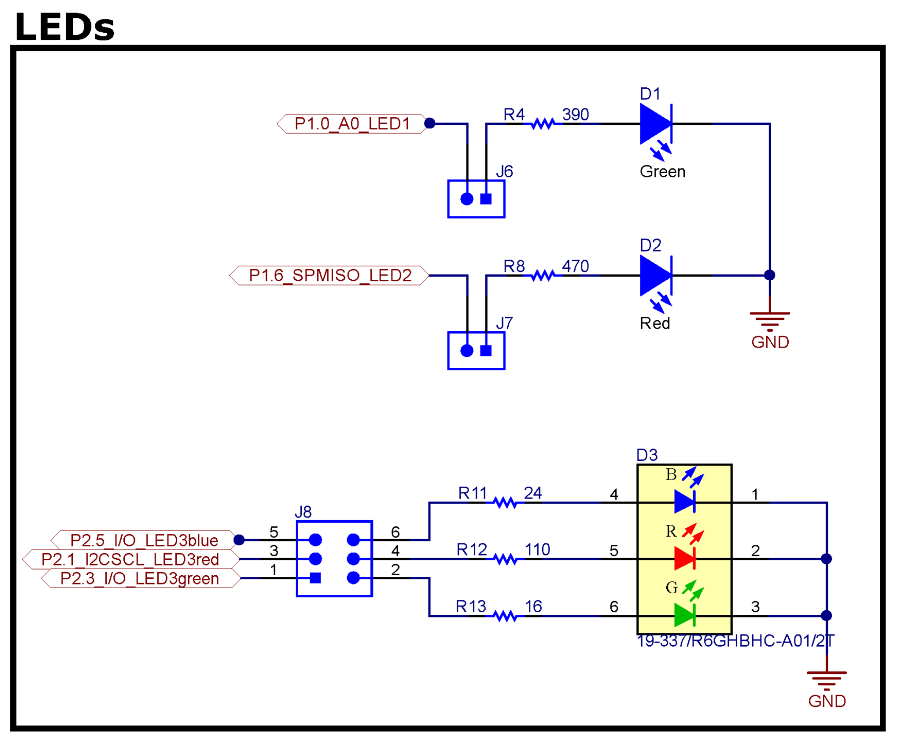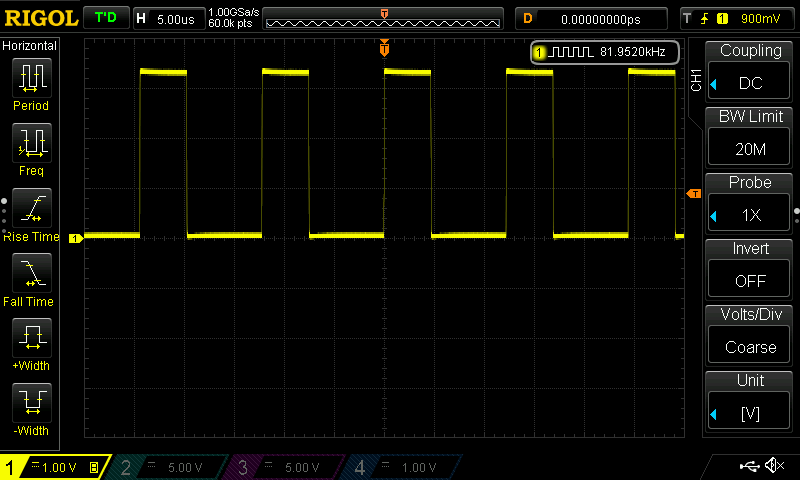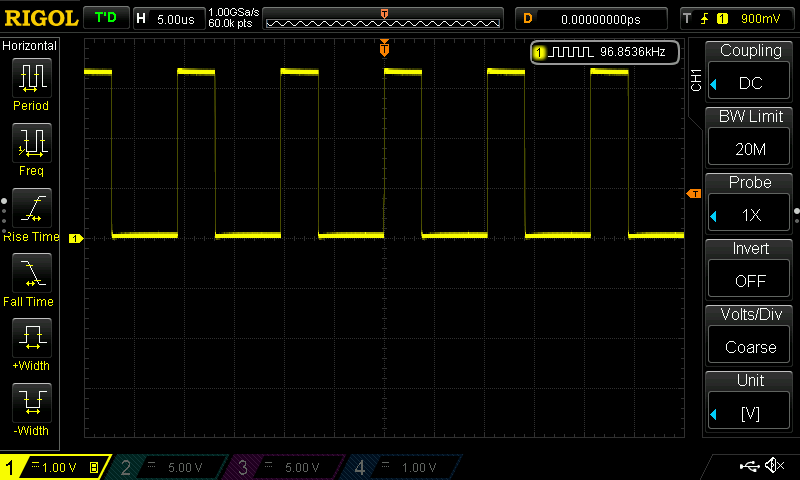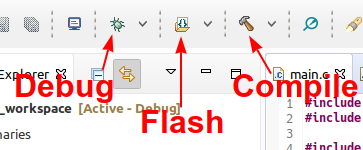# Parallel Interface - Outputs

## First Program

• With the help of outputs of the parallel interface for example LEDs can be switched on and off.
• On the Launchpad there are five LEDs, see the documentation of the Lauchpad• In the first program the red and the green LEDs will blink. Make sure the jumper J6 and J7 are connected.
• The green LED is connected to pin P1.0, the red LED to P1.6
First program - blinking the red and green LED
#include <msp430.h>

int main(void) {
WDTCTL = WDTPW | WDTHOLD;  // stop watchdog timer

P1DIR = 0xFF;
__no_operation();

while (1) {
P1OUT = 0x41;
__no_operation();
P1OUT = 0x00;
__no_operation();
}
}


Line-per-line explanation of the program

• #include <msp430.h>
• Including the MSP430 header file
• Containing the definition of multiple MSP430 register constants, macros, ...
• e. g. WDTCTL, WDTPW, P1DIR, P1OUT, BIT0, BIT1
• int main(void) {
• Head of a typical C main function
• Convention: main function returns an int
• Our main function returns nothing, because we use a endless loop
• Typical approach: microcontroller never end!
• WDTCTL = WDTPW | WDTHOLD;
• Disabling the so-called watchdog timer (WDT) of the MSP430
• This command is mandatory, otherwise WDT will stop the program immediately
• Don't forget to add this command as the first command of every program!
• P1DIR = 0xFF;
• P1DIR is the direction register of all 8 pins of port 1.
• According to the value of a single bit the corresponding pin is set to an input or output.
• The value 0xFF = 0b1111 1111 ensures, that all pins of port 1 are set to output.
• __no_operation();
An additional no-operation command is added to enable a breakpoint after the execution of the previous register change. This command is not needed.
• while (1) {
• P1OUT = 0x41;
• The output register of port 1 P1OUT defines the values of the output pins.
• 0x41 = 0b0100 0001. The bits 0 and 6 are set.
• This results into Pin P1.0 and P1.6 been set H level → both LEDs turn on.
• P1OUT = 0x00;
• Resets all bits of the output register.
• Both LEDs turn off.

What happens inside the MSP430 when setting values to the port registers?Question

1. What happens to the LEDs when the program runs freely?
2. Probe the pins P1.0 and P1.6 of the MSP430 with an oscilloscope. Measure the frequency of the square wave.
3. Why is the duty-cycle of the signal not 50%?
4. Remove all __no_operation() instructions and flash the MSP430 again. What are the changes in the signal?
5. The default clock frequency of the MSP430 is approx. 1 MHz. How many clock cycles does the MSP430 need to run one loop iteration? Per no-operation instruction only one clock cycle is needed.Solution

$n = \frac{f\ped{clock}}{f\ped{signal}}$
$n_1 \approx 12, \quad n_2 \approx 10$

Info

Debugging, Flashing and Compiling in Code Composer Studio• If you want to debug your program:
• using breakpoint, single-step the program, watch register values, ...
• then program the MSP430 by clicking on the green bug symbol.
• If you want to program on the MSP430 without debugging - just let the new program run freely - then press the flash symbol, right to the debug symbol.
• If you want to verify your code, find out syntax error: click the compile symbol

## Delaying the Program

### Using a Loop

In order to see the LEDs blink the program need to be delayed by a software loops. Multiple possibilities are shown in the following programs.

Delaying the LED blinking using a while loop
#include <msp430.h>
#include <stdint.h>

int main(void) {
WDTCTL = WDTPW | WDTHOLD;  // stop watchdog timer

P1DIR = 0xFF;

while (1) {
P1OUT = 0x41;

volatile uint16_t counter = 50000;
while (counter > 0) {
counter--;
}

P1OUT = 0x00;
counter = 50000;
while (counter > 0) {
counter--;
}
}
}


#### C Data Types

• data types in C for integers: char, short, int, long
• prefix unsigned make variable unsigned, the value range can be doubled.
• for ASCII character char is used too.
• native data types for boolean and character strings are not implemented.
• data type int is typically one word wide. In case of the MSP430 16 bit, on a PC 32 bit.
• More uniform is usage of the data types defined in the header file stdint.h.
#include <stdint.h>
stdint native size minimum maximum
int8_t char 8 bit -128 127
uint8_t unsigned char or byte 8 bit 0 255
int16_t short (int) 16 bit -32 768 32 767
uint16_t unsigned short (unsigned int) 16 bit 0 65 535
int32_t long 32 bit -2 147 483 648 2 147 483 647
uint32_t unsigned long 32 bit 0 4 294 967 295

#### Volatile Variables and Compiler Optimization

• With the declaration of the variable counter the keyword volatile is added.
• This keyword ensures that the compiler does no optimization when working with the variable.
• Removing the keyword volatile results into the loops being optimized and removed. No delay is added.
• Alternatively the complier optimization can be disabled in the compiler options.

Question

Use 10000 iterations as the delay. Ensure the doubled delay time is applied correctly.

### Using a Function

Delaying the LED blinking using a function
#include <msp430.h>
#include <stdint.h>

void delay(uint16_t duration);

int main(void) {
WDTCTL = WDTPW | WDTHOLD;  // stop watchdog timer

P1DIR = 0xFF;

while (1) {
P1OUT = 0x41;
delay(50000);
P1OUT = 0x00;
delay(50000);
}
}

void delay(uint16_t duration) {
volatile uint16_t counter = duration;
while (counter > 0) {
counter--;
}
}

• void delay(uint16_t duration);
• This line is the prototype of the function.
• Every function needs an prototype so, that the C code can call this function.
• The prototype line is identical to function head, except a semicolon on the end.
• void delay(uint16_t duration) {
• This line is the head of the function.
• The return type is void (nothing).
• The function name is delay.
• The function need one parameter of type uint16_t called duration.
• The function body is followed.
• ... structuring and organizing your program.

### Using __delay_cycles()

• __delay_cycles(n) is a built-in macro of the msp430.h.
• The macro creates a delay of exactly n clock cycles.
• n needs to be an known value while compiling the program otherwise the will be a compile error.
Delaying the LED blinking using __delay_cycles()
#include <msp430.h>
#include <stdint.h>

int main(void) {
WDTCTL = WDTPW | WDTHOLD;  // stop watchdog timer

P1DIR = 0xFF;

while (1) {
P1OUT = 0x41;
__delay_cycles(500000);
P1OUT = 0x00;
__delay_cycles(500000);
}
}


## Bit Manipulation

Info

Bit Manipulation

• Setting multiple bits in a variable: variable |= bits;
• Resetting multiple bits in a variable: variable &= ~bits;
• Toggling multiple bits in a variable: variable ^= bits;

Bit Constants
The following bit constants are defined in the msp430.h.

Constant Bin Hex Dec
BIT0 0000 0001 0x01 1
BIT1 0000 0010 0x02 2
BIT2 0000 0100 0x04 4
BIT3 0000 1000 0x08 8
BIT4 0001 0000 0x10 16
BIT5 0010 0000 0x20 32
BIT6 0100 0000 0x40 64
BIT7 1000 0000 0x80 127

Question

Whats the registers value after the execution of the command? Try to calculate the values by your own.

Register's Value Command Result
P1DIR = 0; P1DIR \|= 0b01000001;
P1DIR = 0xF1; P1DIR \|= 0b01000001;
P1OUT = 0xA5; P1OUT &=~ 0b00000001;
P1OUT = 0xA5; P1OUT ^= 0b00001111;
x = 0; x |= BIT0 + BIT6;
y = 0x55; y &= ~(BIT0 + BIT2);
P1OUT = 0x40; P1OUT ^= BIT0 + BIT6

Check your results by e.g. using the Python Online Shell: https://www.python.org/shell/. You can use the following command structure to see the results.
P1DIR = 0; P1DIR |= 0b01000001; print(hex(P1DIR) + " " + bin(P1DIR))

#include <msp430.h>
#include <stdint.h>

int main(void) {
WDTCTL = WDTPW | WDTHOLD;  // stop watchdog timer

P1DIR |= BIT0 + BIT6;
P1OUT &= ~(BIT0 + BIT6);

while (1) {
P1OUT |= BIT0;
P1OUT ^= BIT6;
__delay_cycles(500000);
P1OUT &= ~BIT0;
P1OUT ^= BIT6;
__delay_cycles(500000);
}
}

• P1DIR |= BIT0 + BIT6; Setting pins P1.0 and P1.6 to output.
• P1OUT &= ~(BIT0 + BIT6); Resetting both LED pins to GND.
• P1OUT |= BIT0; Set P1.0 to Vcc.
• P1OUT ^= BIT6; Toggle P1.6.
• P1OUT &= ~BIT0; Reset P1.0 to GND.

Question

The pins P2.1, P2.3 and P2.5 are connected to the RGB LED of the Launchpad (see pinout). Write a program that loop through the following colors:

• red
• yellow
• green
• cyan
• blue
• magenta

Solution

#include <msp430.h>
#include <stdint.h>

#define P_RED (BIT1)
#define P_GREEN (BIT3)
#define P_BLUE (BIT5)
#define DELAY (1000000)

int main(void) {
WDTCTL = WDTPW | WDTHOLD;  // stop watchdog timer

P2DIR |= BIT1 + BIT3 + BIT5;
P2OUT &= ~(P_RED + P_GREEN + P_BLUE);

// red
P2OUT |= P_RED;

while (1) {
// red
P2OUT &= ~P_BLUE;
__delay_cycles(DELAY);

// yellow
P2OUT |= P_GREEN;
__delay_cycles(DELAY);

// green
P2OUT &= ~P_RED;
__delay_cycles(DELAY);

// cyan
P2OUT |= P_BLUE;
__delay_cycles(DELAY);

// blue
P2OUT &= ~ P_GREEN;
__delay_cycles(DELAY);

// magenta
P2OUT |= P_RED;
__delay_cycles(DELAY);
}
}